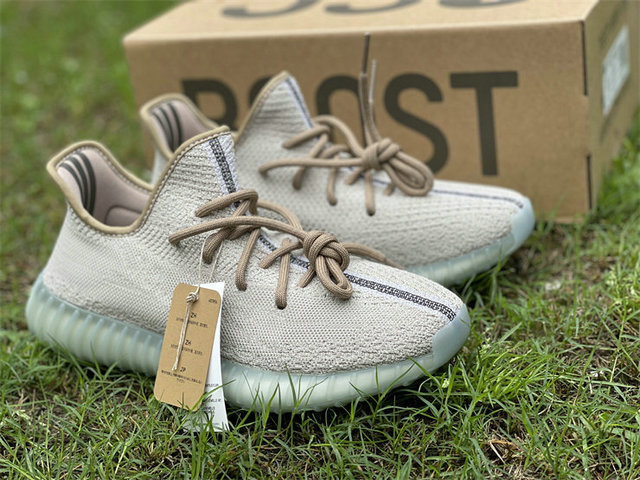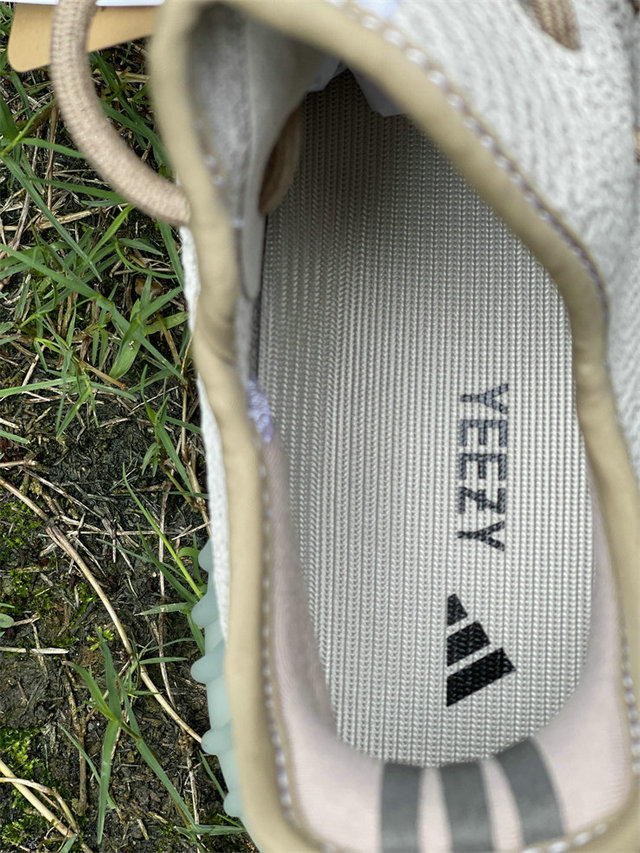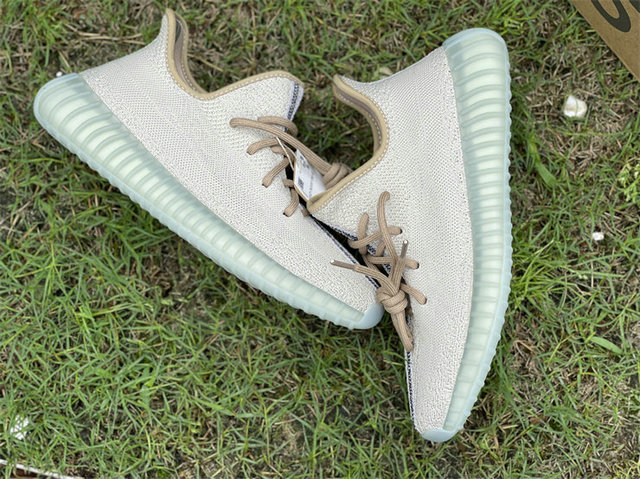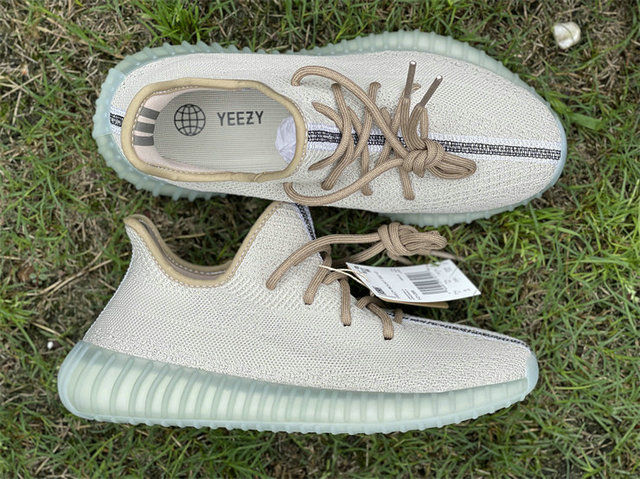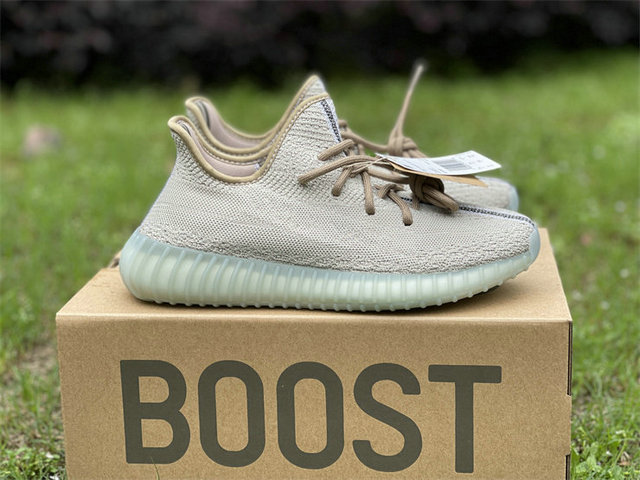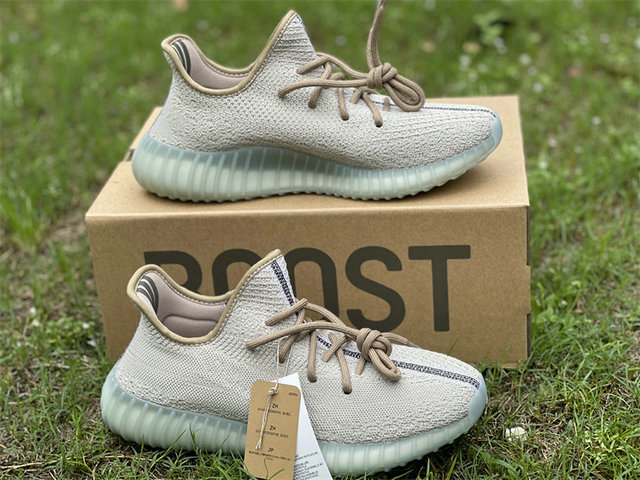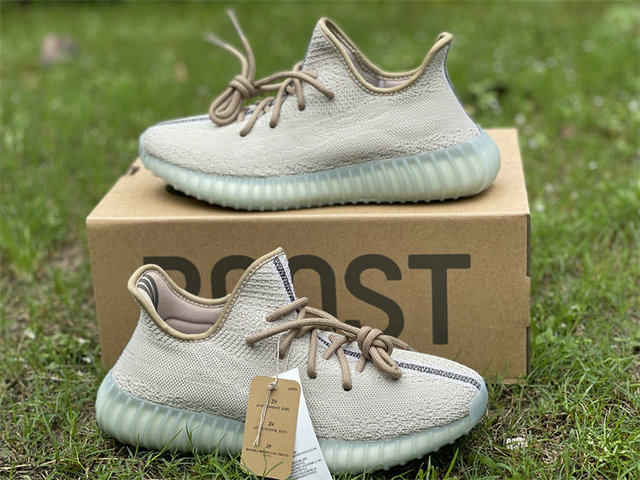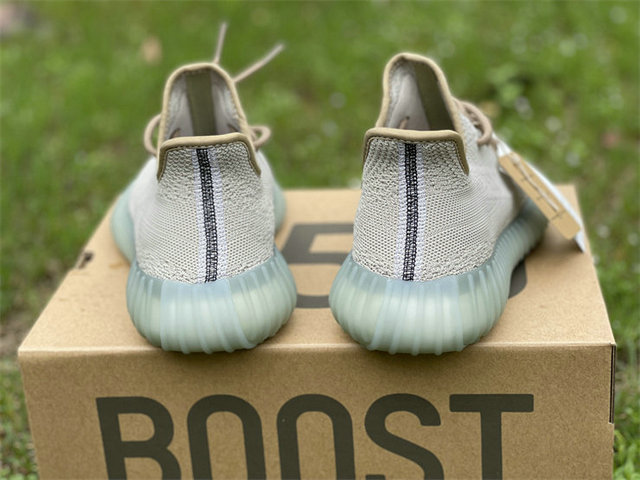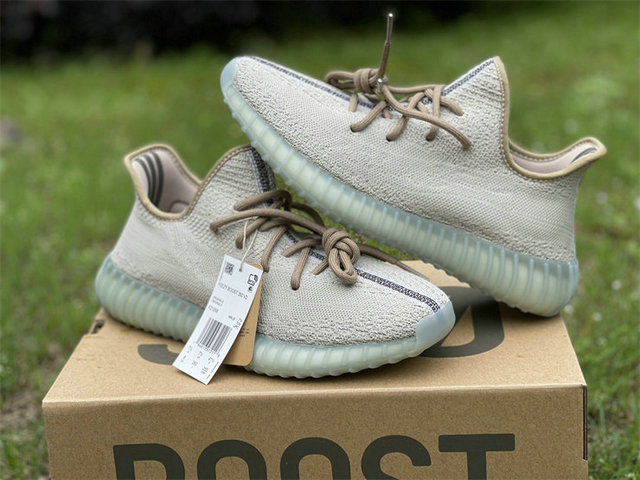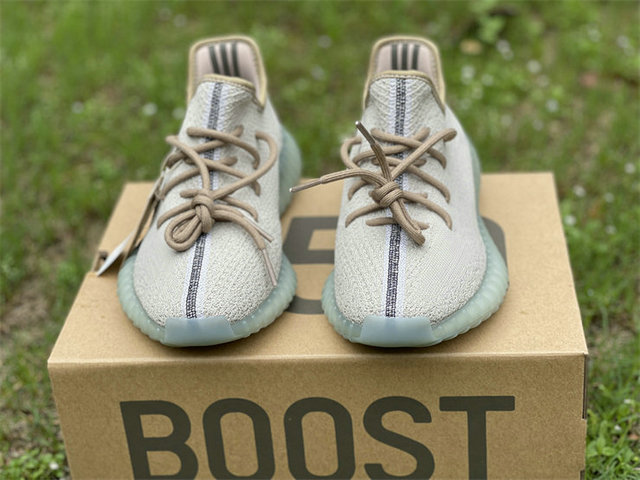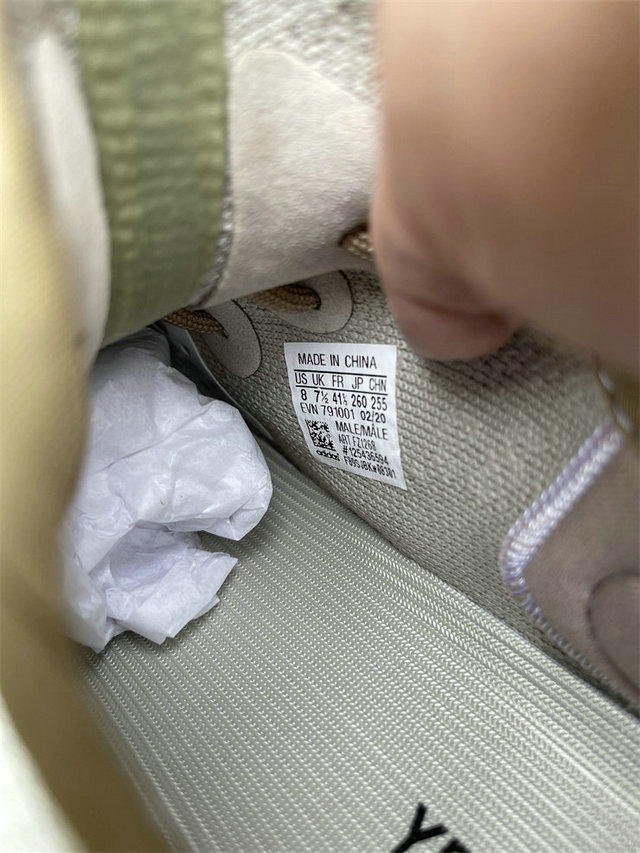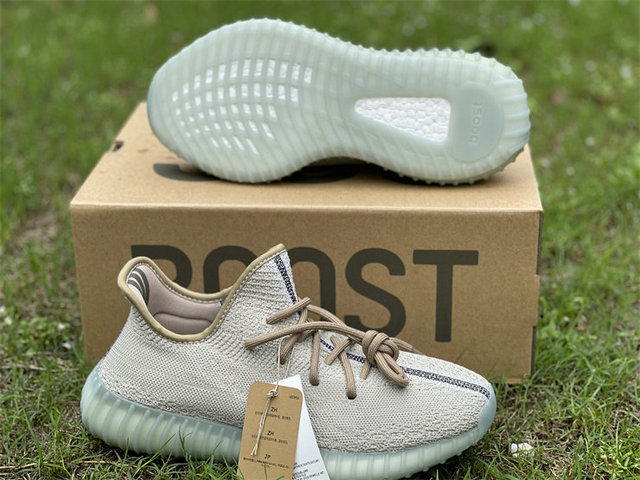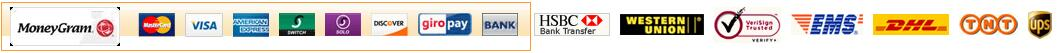USD

# Authentic Y 350 V2 Brown/Green Item NO.: 1602581

Write a Review
US\$ 150.00
Size
US4=UK3.5=Fr 36 US4.5=UK4=Fr 37 1/3 US5=UK4.5=Fr 37 2/3 US5.5=UK5=Fr 38 US6.5=UK6=Fr 39 1/3 US6=UK5.5=Fr 38 2/3 US7=UK6.5=Fr 40 US7.5=UK7=Fr 40 2/3 US8=UK7.5=Fr 41 1/3 US8.5=UK8=Fr 42 US9=UK8.5=Fr 42 2/3 US9.5=UK9=Fr 43 1/3 US10=UK9.5=Fr 44 US10.5=UK10=Fr 44 2/3 US11=UK10.5=Fr 45 1/3 US11.5=UK11=Fr 46 US12=UK11.5=Fr 46 2/3 US12.5=UK12=Fr 47 1/3 US13=UK12.5=Fr 48
Quantity